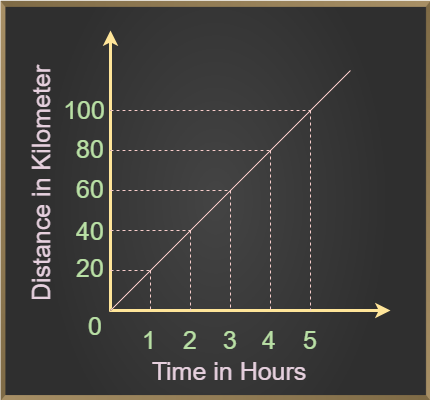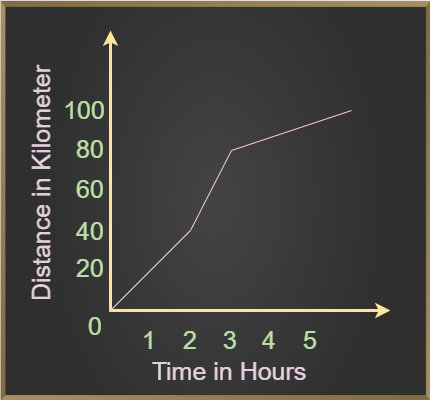# Uniform and Non-Uniform Motion

Uniform and Non-Uniform Motion are two types of motion based on the rate of covering distance per unit of time. In the case of Uniform Motion, the rate of covering distance per unit time is the same while in the case of Non-Uniform Motion, the rate of covering distance per unit time is different. “Motion of a Body” is defined as the change in the position of the body with respect to the time taken. Some examples of objects exhibiting motion are a moving bus, the hands of a clock, the needle of a sewing machine, a cyclist cycling in a park, etc. Any object can majorly perform two types of motion which are uniform motion and non-uniform motion.

In this article, we will learn about Uniform Motion, Non-Uniform Motion, Graph of Uniform Motion, Graph of Non-Uniform Motion, and others in detail.

## Uniform Motion

Uniform Motion of a body can be defined as the motion of a body that travels with constant velocity along a straight line and also covers equal distances in equal intervals of time. As the body is moving in rectilinear motion with a constant velocity, the acceleration of the body is zero.

### Uniform Motion Graph

We can study the uniform motion graph with the help of the example discussed below,

Examples: Let us suppose that a car is travelling at a speed of 20 km/hr, which means the car covers 20 km in one hour. Hence, the speed of the car is constant for every hour and its acceleration is zero. The graph of uniform motion is given below,### Examples of Uniform Motion

Various examples of Uniform Motion are discussed below:

• An airplane cruising at a constant speed.
• A car is going along a straight road at a constant speed.
• A train at constant speed moving along straight tracks.

## Non-Uniform Motion

The non-uniform motion of a body is defined as the motion of a body that travels at odd speeds and doesn’t cover equal distances at equal time intervals, irrespective of the duration of time. As the body is moving in rectilinear motion and the motion of the body is not consistent, the acceleration of the body will be non-zero.

### Non-Uniform Motion Graph

We can study the non-uniform motion graph with the help of the example discussed below,

Examples: A cyclist travelled 40 km in the first 2 hours and next 40 km in 1 hour and the last 20 km in 2 hours. This shows that the speed of the cyclist is inconsistent and he is in non-uniform motion. The graph of Non-Uniform Motion is given below,### Examples of Non-Uniform Motion

The majority of the motions around us are non-uniform in nature. Various examples of Non-Uniform Motion are discussed below:

• A ball bouncing randomly
• A horse running a race
• A car accelerating along the curve path
• Movement of an asteroid

## Difference between Uniform and Non-Uniform Motion

The difference between Uniform and NonUniformotion can be understood with the help of the table given below

Uniform motion

Non-uniform motion

In uniform motion, an object covers equal distances at equal intervals of time. In a non-uniform motion, the object does not cover equal distances at equal intervals of time.
The average speed of the object during the whole journey is equal to its actual speed in uniform motion. The average speed of the object during the whole journey is different from its actual speed in non-uniform motion.
Distance-time graph of an object with uniform motion is a straight line. Distance-time graph of an object with non-uniform motion is a curved line.
Acceleration of the object is zero in Uniform Motion. Acceleration of the object will be non-zero in Non-Uniform Motion.

## Uniform and Non-Uniform Circular Motion

Uniform circular motion of an object is the motion of an object that is moving in circular path and has a fixed speed. Whereas, Non-Uniform Circular Motion is the motions of the object in circular motion with variable speed. Let’s learn about them in detail.

## What are Uniform Circular Motions?

A body is said to be in a uniform circular motion if it moves at constant speed around a fixed axis or point, the object in uniform circular motion travels in a curved path, and it is at a constant radial distance from the centre point at any given time, and its velocity is tangential to the curved path.

## What are Non-Uniform Circular Motions?

A body is said to be in the state of non-uniform circular motion if it travels at variable angular speed around a fixed axis or centre point, here the motion of that object is inconsistent as the object covers an unequal distance in unequal time intervals.

Also, Check

## Uniform and Non-Uniform Motion – FAQs

### 1. What is Uniform Motion?

When a body travels with constant velocity along a straight line and covers equal distances in equal intervals of time, irrespective of the time duration, the body is said to be in Uniform Motion and the acceleration of the body is zero.

Example: A car moving at constant speed along the highway.

### 2. What is Non-Uniform Motion?

When a body travels at odd speeds and doesn’t cover equal distances at equal time intervals, irrespective of the duration of time, the body is said to be in non-uniform motion and the acceleration of the body will be non-zero.

Example: Motion of bees in the garden.

### 3. What are Uniform Motion and Non-Uniform Motion Examples?

Example of Uniform Motion are:

• Motion of Pendulum in space
• Motion of Plane cruising at a constant speed

Example of Non-Uniform Motion are:

• Motion of fish in the river
• Motion of Asteroids

### 4. What is Difference between Uniform and Non-Uniform Motion?

The basic difference between the uniform and the uniform system is that in uniform motion, the velocity of the body is constant whereas in non-uniform motion the velocity of the body is not constant.

### 5. Define Uniform Circular Motion.

Uniform Circular Motion is the motion of an object about a fixed point with constant velocity. For example motion of satellites around a planet represent the uniform circular motion.

Whether you're preparing for your first job interview or aiming to upskill in this ever-evolving tech landscape, GeeksforGeeks Courses are your key to success. We provide top-quality content at affordable prices, all geared towards accelerating your growth in a time-bound manner. Join the millions we've already empowered, and we're here to do the same for you. Don't miss out - check it out now!

Previous
Next